# Simultaneous Equations for Circuit Analysis

## Network Analysis Techniques

• #### Question 1

Solve this equation for the value of x:

 x + 5 = 8

How many exact solutions does the above equation have?

Now, determine a few different solutions for the following equation:

 x + y = 8

How many exact solutions does this equation have? Plot this equation’s solutions on the following graph:• #### Question 2

Plot the solutions to the equation y + x = 8 on a graph: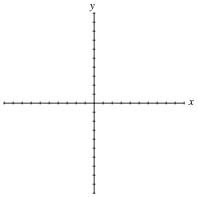On the same graph, plot the solutions to the equation y − x = 3. What is the significance of the point where the two lines cross?

• #### Question 3

What does it actually mean to obtain a solution for a “simultaneous” system of equations? For example, if 2x + y = 7 and x − y = −1, what do the solution values (x = 2 ; y = 3) represent?

If we were to graph both these linear equations on a Cartesian (x, y) coordinate system, where would the solution (2,3) be located on the graph?

• #### Question 4

Plot the equation y = x2 on the following graph:On the same graph, plot the equation y = x + 2. What is the significance of the point where the two plots cross?

• #### Question 5

Load lines are useful tools for analyzing transistor amplifier circuits, but they may be hard to understand at first. To help you understand what “load lines” are useful for and how they are determined, I will apply one to this simple two-resistor circuit: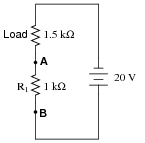We will have to plot a load line for this simple two-resistor circuit along with the “characteristic curve” for resistor R1 in order to see the benefit of a load line. Load lines really only have meaning when superimposed with other plots. First, the characteristic curve for R1, defined as the voltage/current relationship between terminals A and B: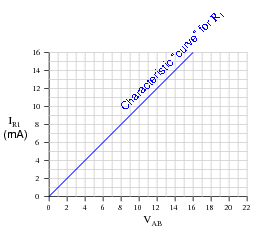Next, I will plot the load line as defined by the 1.5 kΩ load resistor. This “load line” expresses the voltage available between the same two terminals (VAB) as a function of the load current, to account for voltage dropped across the load: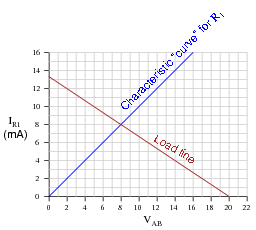At what value of current (IR1) do the two lines intersect? Explain what is significant about this value of current.

• #### Question 6

Load lines are useful tools for analyzing transistor amplifier circuits, but they may be applied to other types of circuits as well. Take for instance this diode-resistor circuit:The diode’s characteristic curve is already plotted on the following graph. Your task is to plot the load line for the circuit on the same graph, and note where the two lines intersect: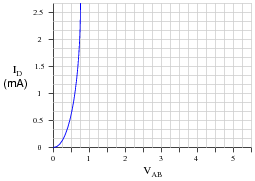What is the practical significance of these two plots’ intersection?

• #### Question 7

Suppose you were given the following two equations and asked to find solutions for x and y that will satisfy both at the same time:

 y + x = 8

 y − x = 3

If we manipulate the second equation so as to solve for y, we will have a definition of y in terms of x that we may use for substitution in the first equation:

 y = x + 3

Show the process of substitution into the first equation, and how this leads to a single solution for x. Then, use that value of x to solve for y, resulting in a solution set valid for both equations.

• #### Question 8

An interesting and useful property in mathematics is the transitive property:

 If a = b and b = c , then a = c

Simply stated, two variables must be equal to one another if they are both equal to a common (third) variable. While not particularly profound or breathtaking in scope, this property is nevertheless useful in solving certain mathematical problems.

Suppose you were given the following two equations and asked to find solutions for x and y that will satisfy both at the same time:

 y + x = 8

 y − x = 3

Manipulate both of these equations to solve for y, and then explain how you could apply the transitive principle to solve for x.

• #### Question 9

Suppose you were given the following two equations and asked to find solutions for x and y that will satisfy both at the same time:

 y + x = 8

 y − x = 3

Now, you know that we may do anything we want to either equation as long as we do the same thing to both sides (on either side of the “equal” sign). This is the basic rule we follow when manipulating an equation to solve for a particular variable. For example, we may take the equation y + x = 8 and subtract x from both sides to yield an equation expressed in terms of y: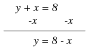Following the same principle, we may take two equations and combine them either by adding or subtracting both sides. For example, we may take the equation y − x = 3 and add both sides of it to the respective sides of the first equation y + x = 8: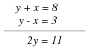What beneficial result comes of this action? In other words, how can I use this new equation 2y = 11 to solve for values of x and y that satisfy both of the original equations?

• #### Question 10

Solve for values of x and y that will satisfy both of the following equations at the same time:

 x + 2y = 9

 4x − y = −18

• #### Question 11

Solve for values of x and y that will satisfy both of the following equations at the same time:

 3x − y = 17

 x + 2y = 1

• #### Question 12

Solve for values of x and y that will satisfy both of the following equations at the same time:

 3x − y = −9

 x 2y = 4

• #### Question 13

If we wish to solve for the value of three inter-related variables (i.e. x y z = 0), how many equations do we need in our “system” of simultaneous equations, total?

Graphically, what does the solution set (x,y,z) represent for a system of equations with three variables?

• #### Question 14

Many circuit analysis techniques require the solution of “systems of linear equations,” sometimes called “simultaneous equations.” This question is really a series of practice problems for solving simultaneous linear equations, the purpose being to give you lots of practice using various solution techniques (including the solution facilities of your calculator).

Systems of two variables:

 x + y = 5 x − y = −6 2x + y = 7

 x − y = 1 2x − y = 4 x − y = 2

 3x − 2y = −1 −10x + 2y = 0 3x − 5y = −13

 5x + y = −6 −3x − 5y = −28 −x + 2y = 5

 1000x − 500y = 0 −15000x + 2200y = −66200 9100x − 5000y = 24

 550x + 2500y = 5550 7900x − 2800y = 28300 −5200x − 2700y = −6.5

Systems of three variables:

 x − y z = 1 3x + 2y − 5z = −21 x + y + z = 0

 −x − y + z = −1 x − 3y + z = 8 2x − y − 4z = −9

 x + y + z = 3 −x − y − z = −12 −2x + 2y − z = 12

 x + y − 2z = −12 −4x − 3y + 2z = −32 19x − 6y + 20z = −33

 3x − 2y + z = 19 x − 2y + 3z = −1 4x + 5y − 3z = −17

 −4x + 3y − 5z = −45 −2x + 7y − z = 3 −7x + 2y − 8z = 9

 890x − 1000y + 2500z = −1500 2750x − 6200y + 4500z = 17500

 3300x + 7200y − 5100z = 21500 −10000x + 5300y − 1000z = 8100

 −x + y − z = 0 6x − 2y − 3z = 5
• #### Question 15

Suppose you needed to choose a fixed resistor value (R) to make a voltage divider circuit, given a known potentiometer resistance value, the source voltage value, and the desired range of adjustment: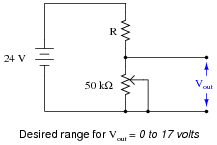Solve for R, and show the equation you set up in order to do it.

Hint: remember the series resistor voltage divider formula . . .

$$V_R = V_{total} (\frac{R}{R_{total}})$$

• #### Question 16

Suppose you needed to choose a potentiometer value (R) to make a voltage divider circuit, given a known fixed resistor value, the source voltage value, and the desired range of adjustment: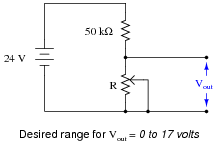Solve for R, and show the equation you set up in order to do it.

Hint: remember the series resistor voltage divider formula . . .

$$V_R = V_{total} (\frac{R}{R_{total}})$$

• #### Question 17

An engineer needs to calculate the values of two resistors to set the minimum and maximum resistance ratios for the following potentiometer circuit: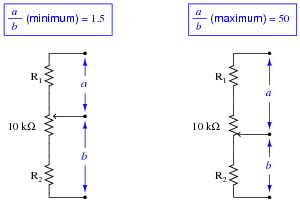First, write an equation for each circuit, showing how resistances R1, R2, and the 10 kΩ of the potentiometer combine to form the ratio [a/b]. Then, use techniques for solving simultaneous equations to calculate actual resistance values for R1 and R2.

• #### Question 18

The voltage gain of a common-emitter transistor amplifier is approximately equal to the collector resistance divided by the emitter resistance: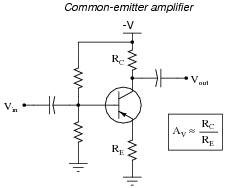Knowing this, calculate the necessary resistance values for the following fixed-value resistor (R2) and potentiometer (R1) to give this common-emitter amplifier an adjustable voltage gain range of 2 to 8:• #### Question 19

The voltage gain of a common-emitter transistor amplifier is approximately equal to the collector resistance divided by the emitter resistance: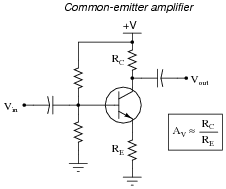Knowing this, calculate the necessary resistance values for the following fixed-value resistors (R1 and R2) to give this common-emitter amplifier an adjustable voltage gain range of 4 to 7: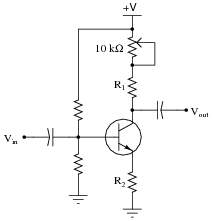• #### Question 20

Suppose you needed to choose two resistance values to make a voltage divider with a limited adjustment range. One of these resistors will be fixed in value (R1), while the other will be variable (a potentiometer connected as a rheostat- R2):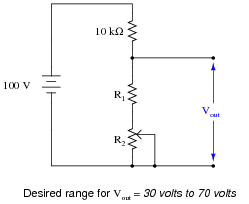Set up a system of simultaneous equations to solve for both R1 and R2, and show how you arrived at the solutions for each.

Hint: remember the series resistor voltage divider formula . . .

$$V_R = V_{total} (\frac{R}{R_{total}})$$

• #### Question 21

Suppose you needed to choose two resistance values to make a voltage divider with a limited adjustment range: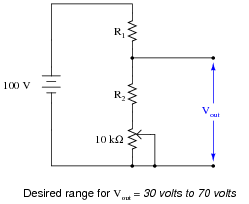Set up a system of simultaneous equations to solve for both R1 and R2, and show how you arrived at the solutions for each.

Hint: remember the series resistor voltage divider formula . . .

$$V_R = V_{total} (\frac{R}{R_{total}})$$

• #### Question 22

Use simultaneous equations to calculate the values of R1 and R2 necessary to give this voltage divider the range of adjustment specified:Vout (minimum) = 3 volts Vout (maximum) = 8 volts

R1 = R2 =

• #### Question 23

Use simultaneous equations to calculate the values of R1 and R2 necessary to give this voltage divider the range of adjustment specified: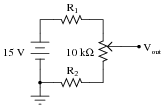Vout (minimum) = 5 volts Vout (maximum) = 12 volts

R1 = R2 =

• #### Question 24

The voltage gain of an inverting operational amplifier circuit is defined by the ratio of feedback to input resistance:

 AV = Rf RiCalculate the necessary values of R1 and R2 to limit the minimum and maximum voltage gain of this opamp circuit to 5 and 30, respectively, given a potentiometer in the middle with a full-span resistance of 5 kΩ:• #### Question 25

Calculate the necessary values of R1 and R2 to limit the minimum and maximum voltage gain of this opamp circuit to 10 and 85, respectively: